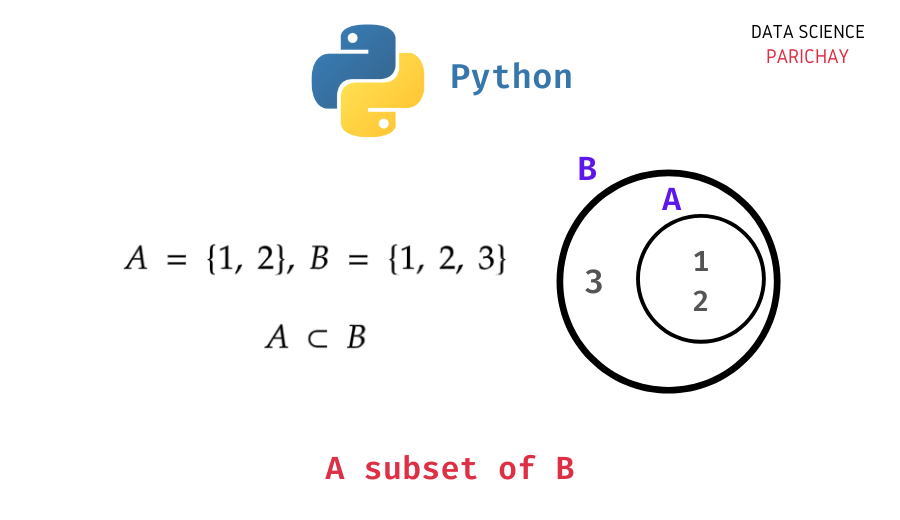# Python – Check if Set is a subset of another Set

In this tutorial, we will look at how to check whether a set in Python is a subset of another set with the help of some examples.

Let’s say we have two sets, A and B. Now, if all the elements of set A are present in set B then A is said to be a subset of B. Here’s an example –

You can see that all the elements of set A are present in set B, thus, we can say that set A is a subset of set B. However, the converse is not true, B is not a subset of A since there are elements in B that are not in A.

The Python set data structure comes with a number of built-in functions to accomplish common set operations like union, intersection, difference, etc. You can use the Python set `issubset()` function to check whether a set is a subset of another set. The following is the syntax:

```# check if a is a subset of b
a.issubset(b)```

We call the `issubset()` function from set a and pass the set b as an argument to check whether set a is a subset of set b. It returns a boolean value. Let’s look at an example.

```# create two sets
a = {1, 2, 3}
b = {1, 2, 3, 4}
# check if a is subset of b
a.issubset(b)```

Output:

`True`

We get `True` as the output since a is in fact, a subset of set b.

Let’s look at another example.

📚 Data Science Programs By Skill Level

Introductory

Intermediate ⭐⭐⭐

🔎 Find Data Science Programs 👨‍💻 111,889 already enrolled

Disclaimer: Data Science Parichay is reader supported. When you purchase a course through a link on this site, we may earn a small commission at no additional cost to you. Earned commissions help support this website and its team of writers.

```# create two sets
a = {1, 2, 3}
b = {1, 3, 4, 5}
# check if a is subset of b
a.issubset(b)```

Output:

`False`

Here we get `False` as the output because not all elements of set a are present in set b (2 from set a is not present in b). Thus, set a is not a subset of set b.

Alternatively, you can use the `<=` operator to check if a set is a subset of another set. For example,

```# create two sets
a = {1, 2, 3}
b = {1, 2, 3, 4}
# check if a is subset of b
a <= b```

Output:

`True`

We get the same result as we did with the set `issubset()` function.

There are also some special cases to keep in mind when checking for subsets.

• Every set is a subset of itself.
```# create a set
a = {1, 2, 3}
# check if a is subset of itself
a.issubset(a)```

Output:

`True`
• Empty set is subset of every set.
```# create a set
a = set()
b = {1, 2, 3}
# check if a is subset of b
a.issubset(b)```

Output:

`True`

You might also be interested in –

•